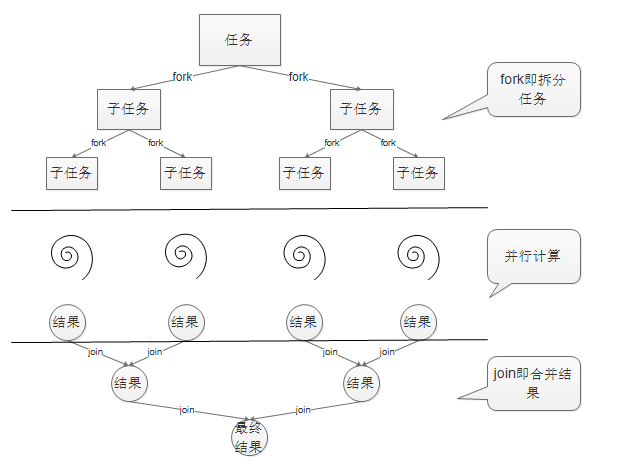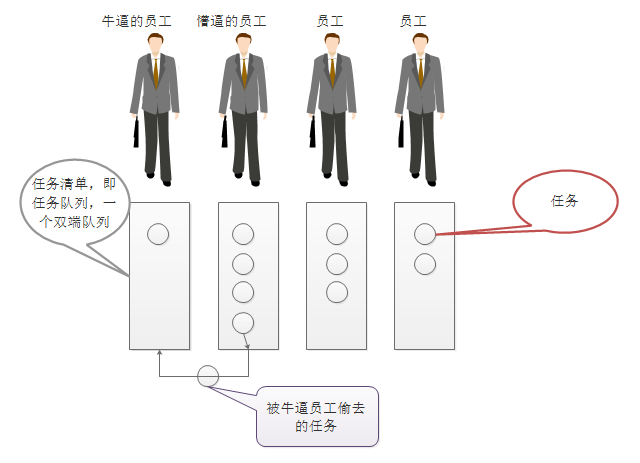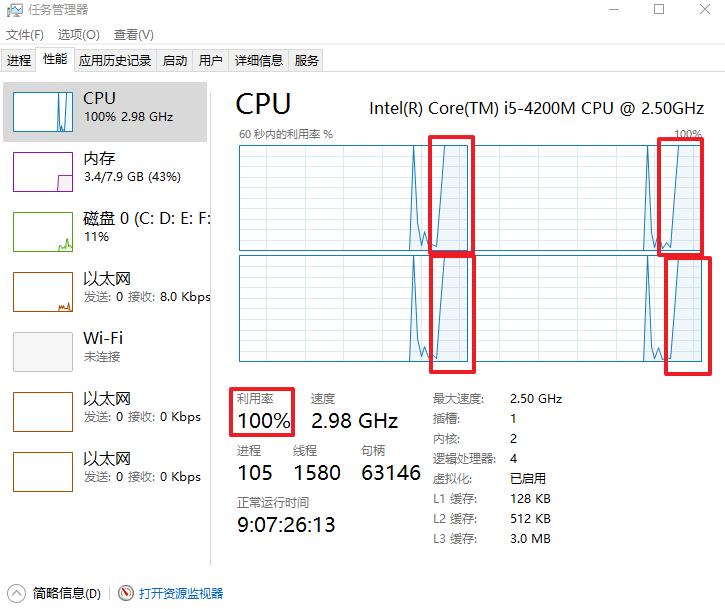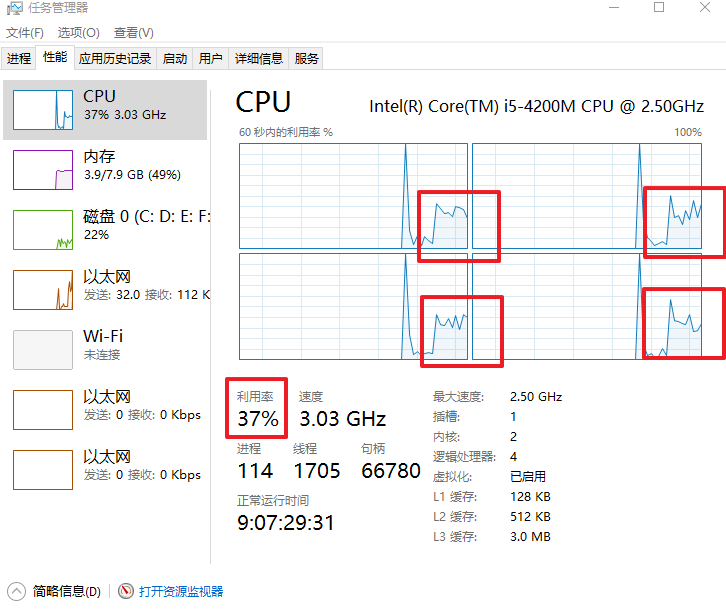# Fork/Join框架介绍

Fork/Join框架是Java 7提供了的一个用于并行执行任务的框架， 大概是怎样子的呢，就是一个把大任务分割成若干个小任务，最终把每个小任务结果汇总起来得到大任务结果的框架。有点像是归并排序。下面的图就能很好地体现出来# 工作窃取模式(work-stealing)# 使用Fork/Join框架



public class ForkJoinCalculator extends RecursiveTask<Long> {

private long start;
private long end;
private static final long THRESHOLD = 10000;// 临界值

public ForkJoinCalculator(long start, long end) {
this.start = start;
this.end = end;
}

@Override
protected Long compute() {

if (end - start <= 1000) {
// 不大于临界值直接计算和
long sum = 0;
for (long i = start; i <= end; i++) {
sum += i;
}
return sum;
} else {
// 大于临界值继续进行拆分子任务
long mid = (start + end) / 2;

// 拆分子任务
ForkJoinCalculator calculator1 = new ForkJoinCalculator(start, mid);
calculator1.fork();

// 拆分子任务
ForkJoinCalculator calculator2 = new ForkJoinCalculator(mid + 1, end);
calculator2.fork();

//合并子任务结果
return calculator1.join() + calculator2.join();
}
}
}


@Test
public void test() {
ForkJoinPool forkJoinPool = new ForkJoinPool();
Long sum = forkJoinPool.invoke(new ForkJoinCalculator(1, 1000000000L));
System.out.println(sum);
}

500000000500000000


# Fork/Join框架的性能

import java.util.concurrent.ForkJoinPool;

import org.junit.Test;

public class ForkJoinTest {

@Test
public void test() {
long start = System.currentTimeMillis();

ForkJoinPool forkJoinPool = new ForkJoinPool();
Long sum = forkJoinPool.invoke(new ForkJoinCalculator(1, 1000000000L));
System.out.println(sum);

long end = System.currentTimeMillis();
System.out.println(end - start);
}

@Test
public void test1() {
long start = System.currentTimeMillis();

long sum = 0;
for (long i = 1; i <= 1000000000L; i++) {
sum += i;
}
System.out.println(sum);

long end = System.currentTimeMillis();
System.out.println(end - start);
}

}test1()方法执行时是这样子的，说明并没有充分利用到CPU05-104737
10-0491208-081万+
01-122566
10-222830
08-01228
03-3138
06-191万+
07-30496
04-22201